You are currently offline. Some features of the site may not work correctly.

# Erdős–Szekeres theorem

Known as: Erdős-Szekeres bound, Erdos-Szekeres theorem, Erdos szekeres theorem
In mathematics, the Erdős–Szekeres theorem is a finitary result that makes precise one of the corollaries of Ramsey's theorem. While Ramsey's theorem… Expand
Wikipedia

## Papers overview

Semantic Scholar uses AI to extract papers important to this topic.
2018
2018
• 2018
• Corpus ID: 119172933
We show a cyclic permutation analogue of Erd\H{o}s-Szekeres Theorem. In particular, we show that every cyclic permutation of… Expand
•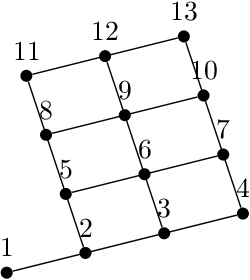Is this relevant?
2014
2014
• 2014
• Corpus ID: 119641212
Abstract The only known skew-translation generalised quadrangles (STGQ) having order ( q , q ) , with q even, are translation… Expand
•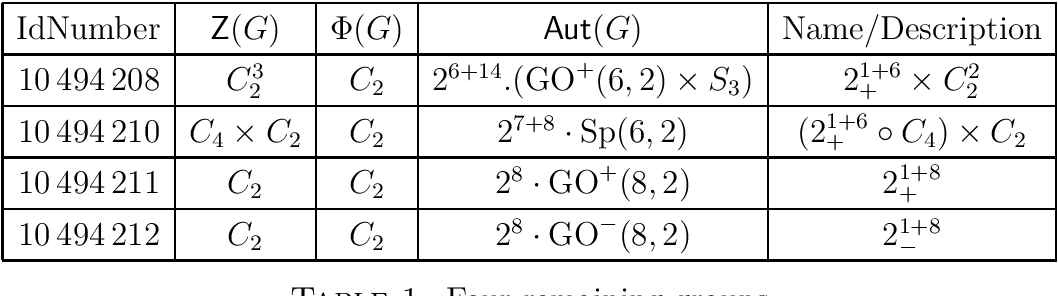Is this relevant?
2013
2013
• Discret. Comput. Geom.
• 2013
• Corpus ID: 20505739
According to the Erdős–Szekeres theorem, for every n, a sufficiently large set of points in general position in the plane… Expand
Is this relevant?
2011
2011
• SoCG '12
• 2011
• Corpus ID: 6400392
Let P=(p1,p2,...,pN) be a sequence of points in the plane, where pi=(xi,yi) and x12...xN. A famous 1935 Erdos-Szekeres theorem… Expand
•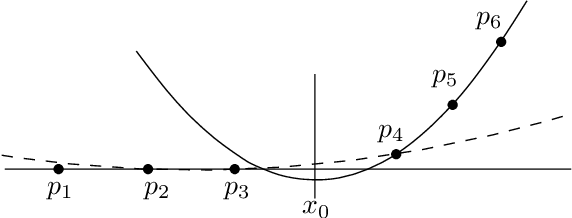•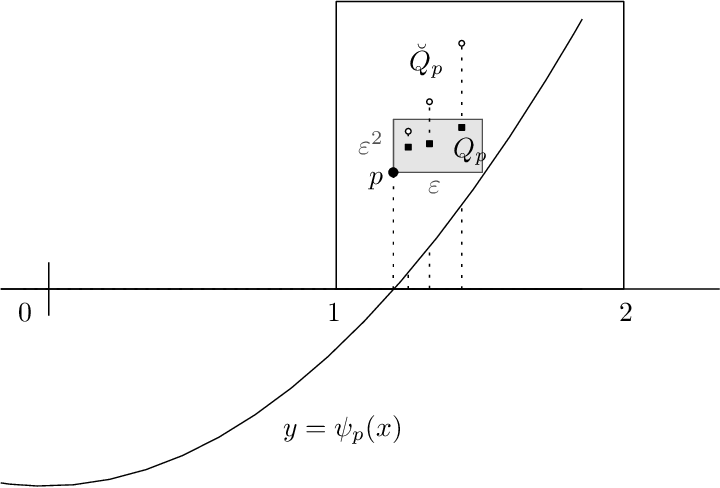•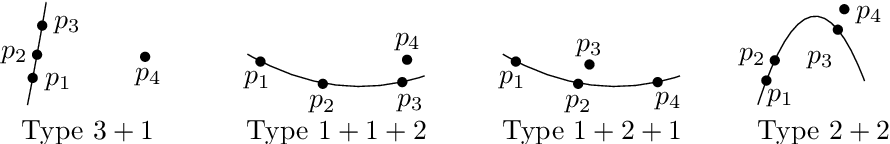Is this relevant?
Highly Cited
2007
Highly Cited
2007
Let P be a finite set of points in general position in the plane. Let C(P) be the convex hull of P and let CiP be the ith convex… Expand
•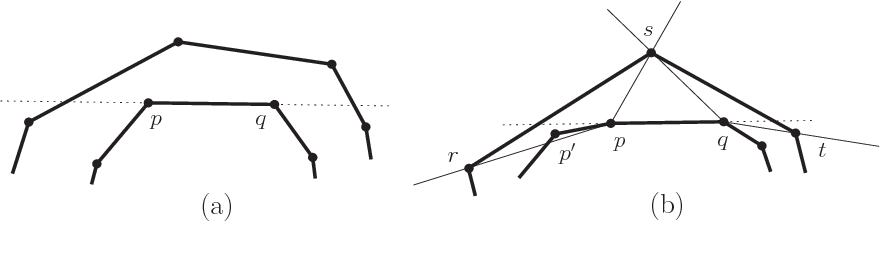•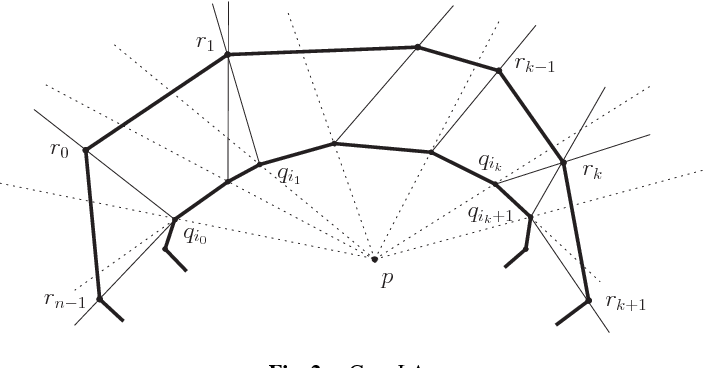•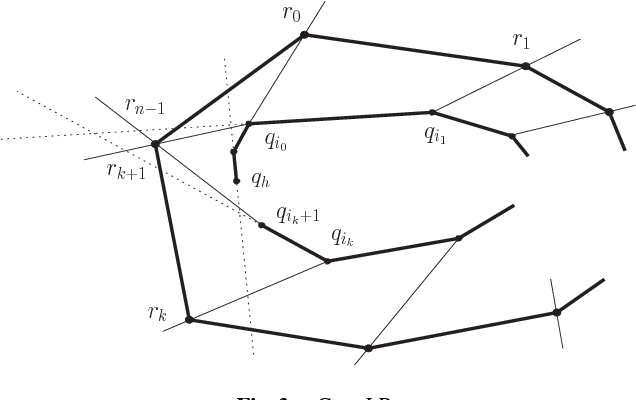•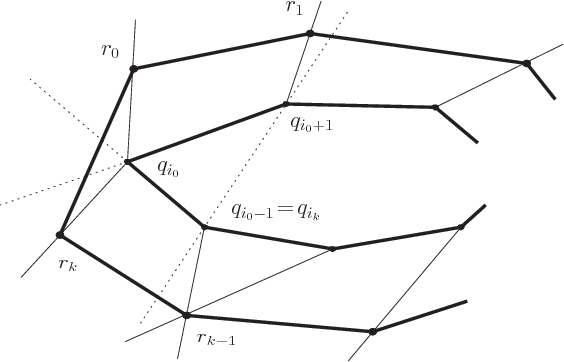Is this relevant?
2006
2006
• J. Comb. Theory, Ser. A
• 2006
• Corpus ID: 17072046
According to the classical Erdos-Szekeres theorem, every sufficiently large set of points in general position in the plane… Expand
•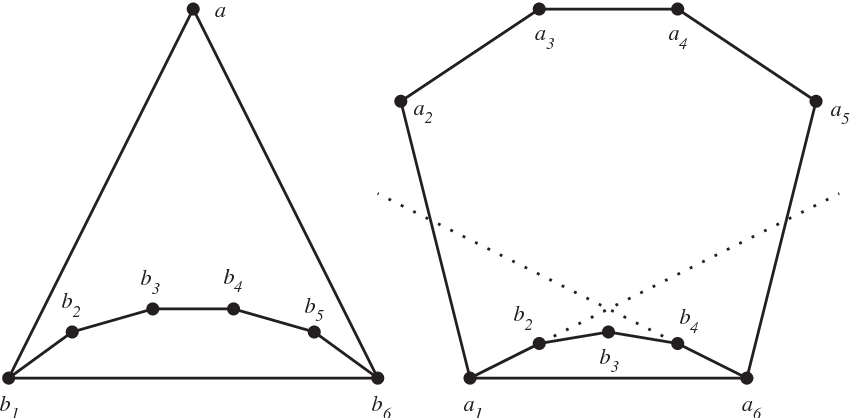•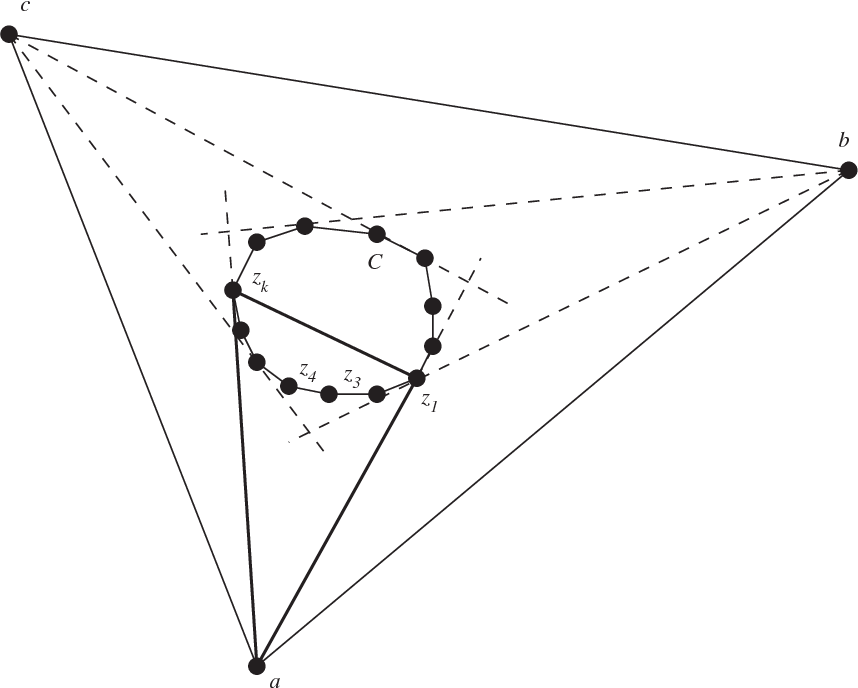•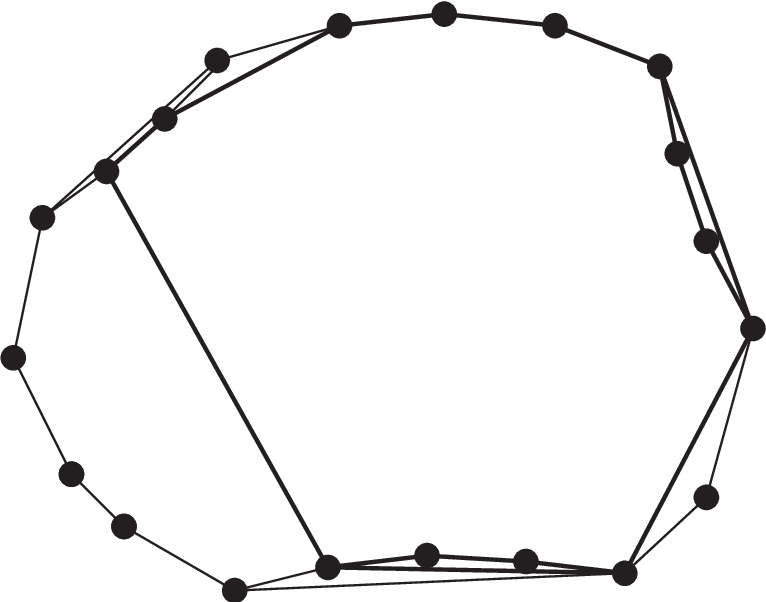•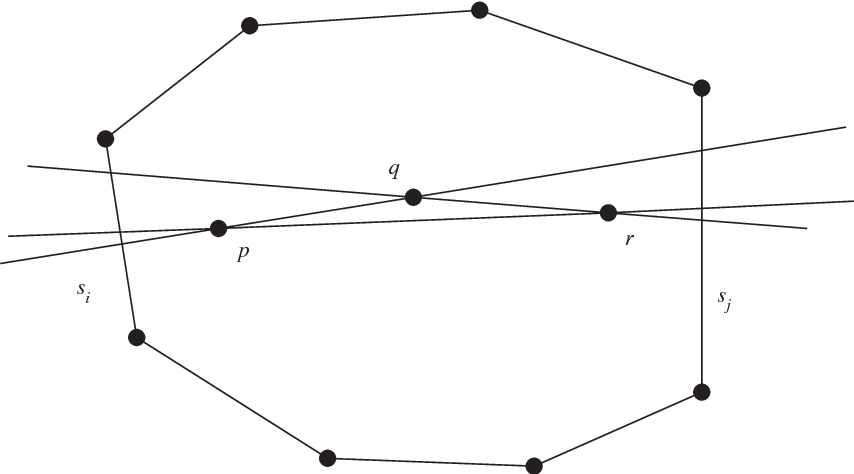Is this relevant?
2004
2004
Combin. Theory (A) 42 (1986) 15–30. 6. D. Foata, Enumerating k-trees, Discrete Math. 1 (1971) 181–186. 7. J. Hage, Enumerating… Expand
Is this relevant?
2002
2002
• 2002
• Corpus ID: 921912
Strong field (exact) solutions of the gravitational field equations of general relativity in the presence of a cosmological… Expand
•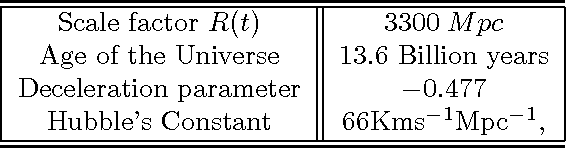•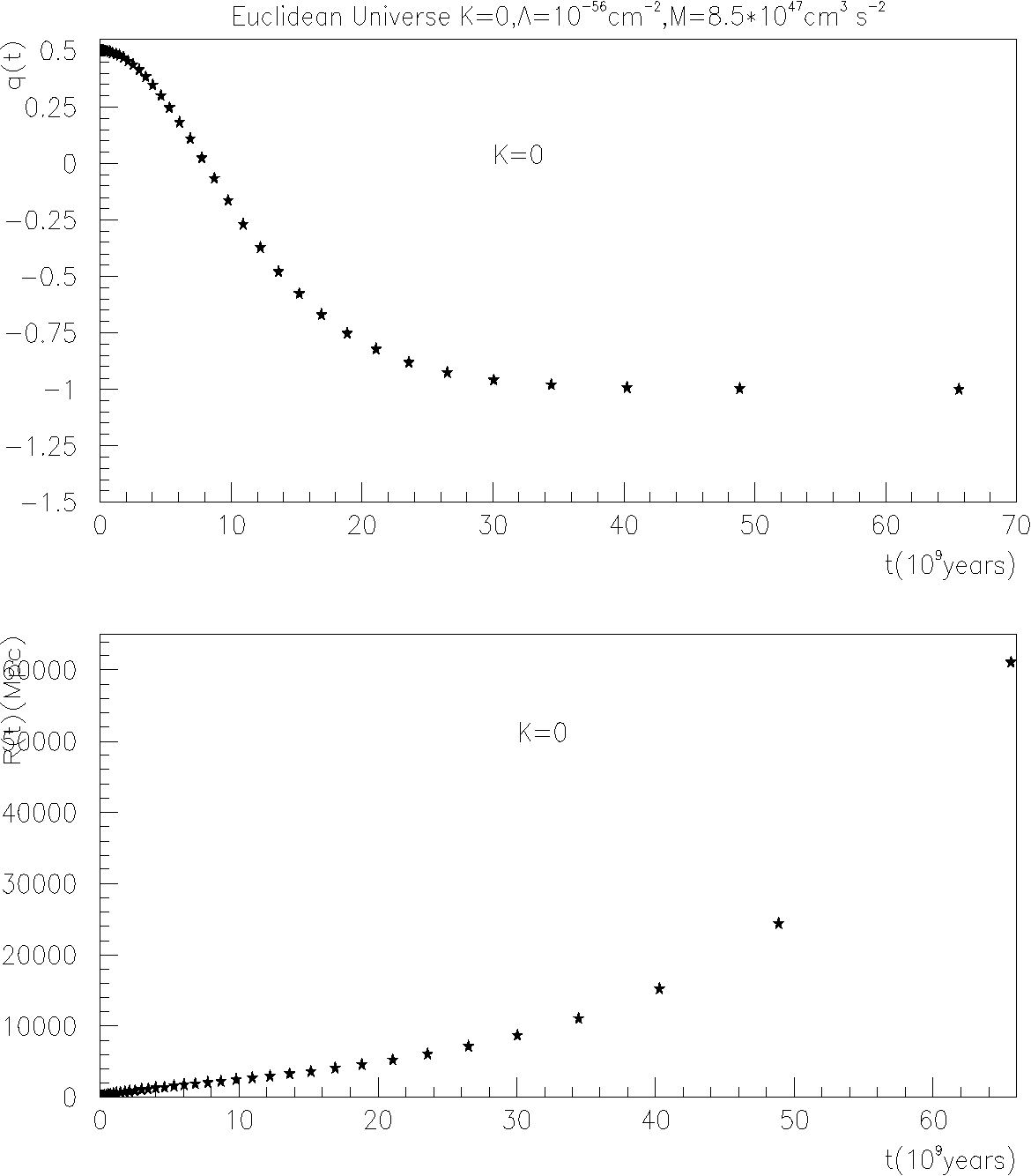•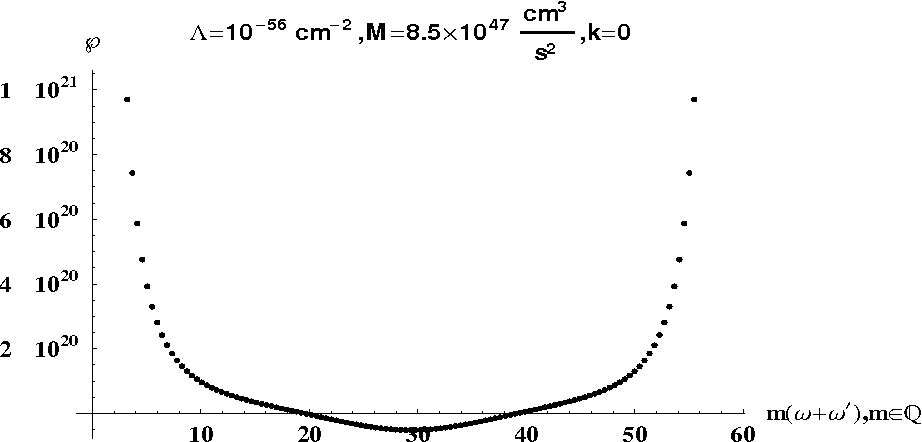•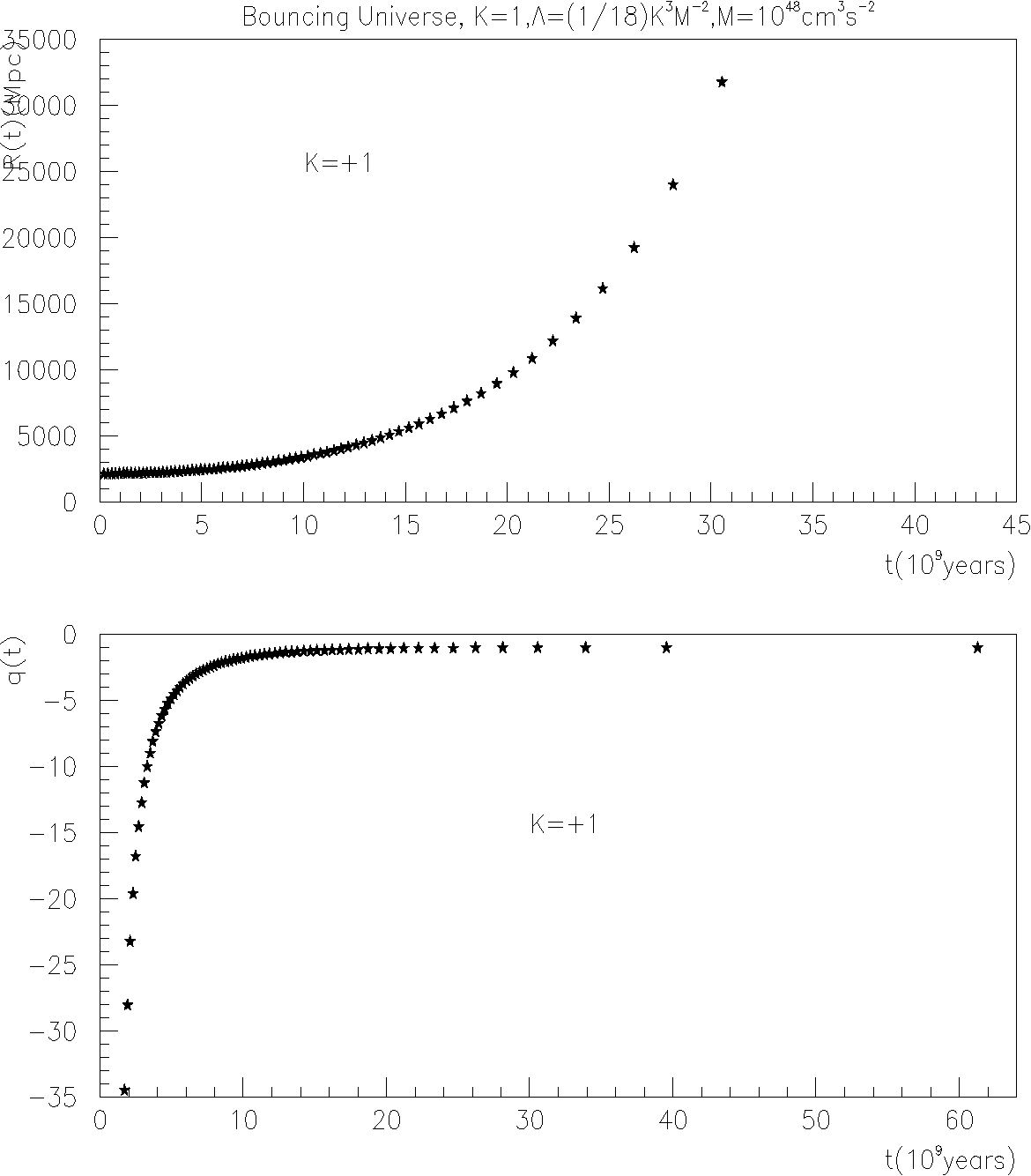•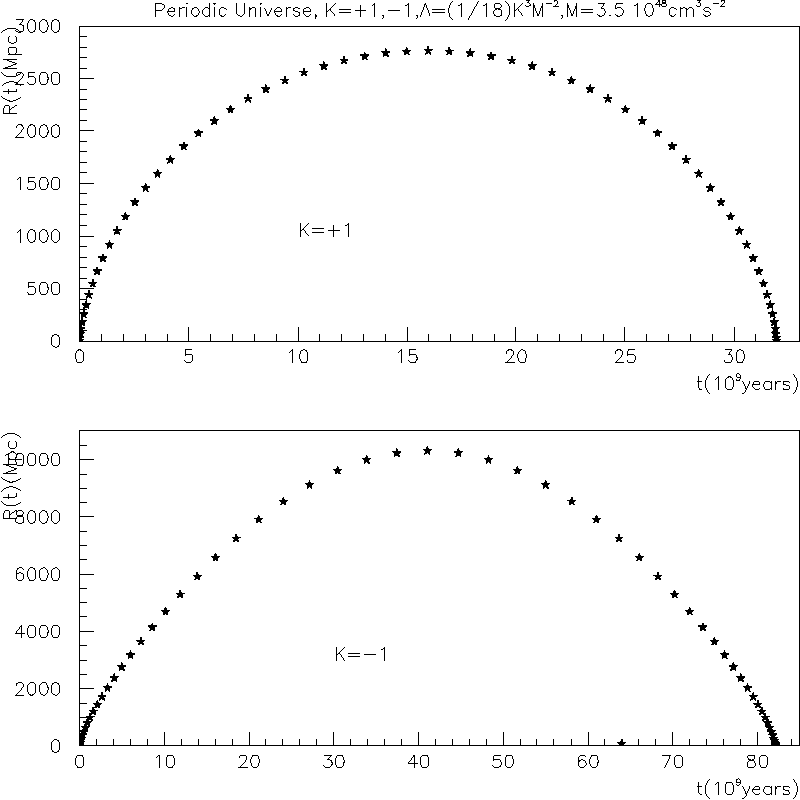Is this relevant?
2001
2001
Abstract As a consequence of the Erdős–Szekeres theorem we prove that, for n large enough, any set of kn points, in general… Expand
•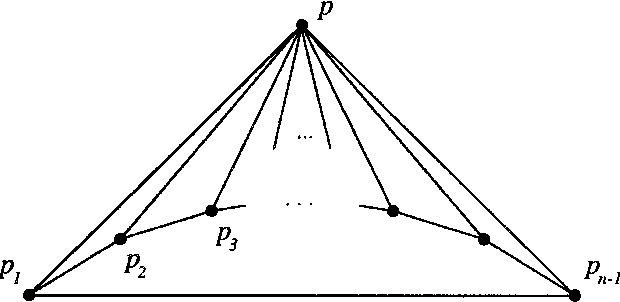•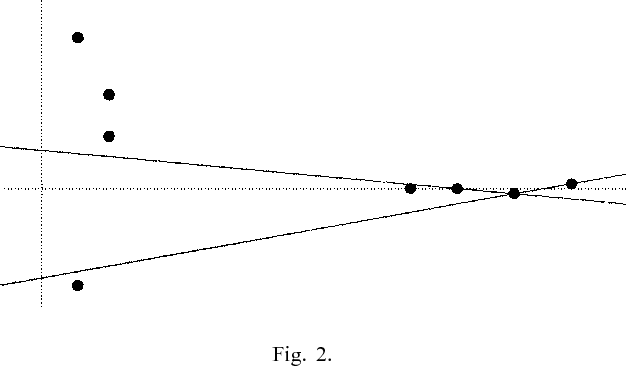•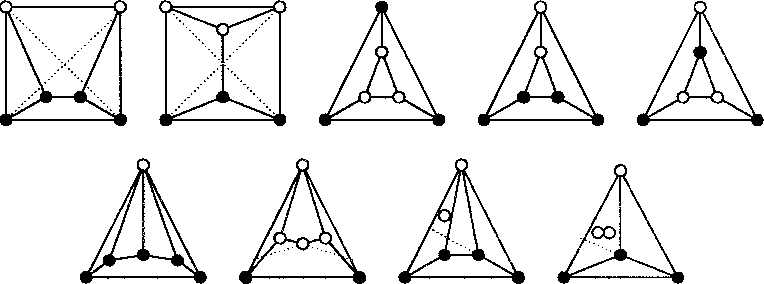•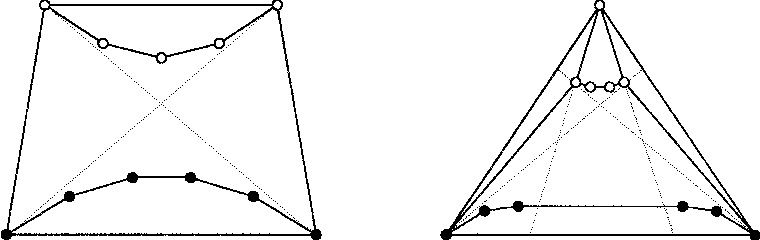Is this relevant?
2000
2000
• JCDCG
• 2000
• Corpus ID: 6460371
Eszter Klein’s theorem claims that among any 5 points in the plane, no three collinear, there is the vertex set of a convex… Expand
Is this relevant?